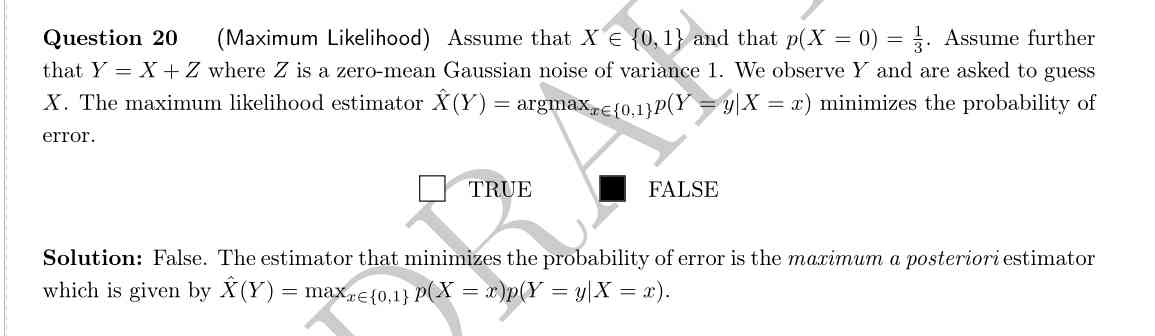### MLE vs MAP

Dear TAs,

If we had no prior p(X=0)=1/3, could we say that the estimator that minimizes the probability is the MLE? Or would the correct answer be MAP estimator with uniform prior?

If the MAP estimator minimizes the probability of error, when would we use MLE? Only when there is no prior? Is there an advantage of using MLE estimator instead of MAP? Is there something that MLE does better?